# Overunity proof article

Proof of overunity efficiency in a R-L circuit with switched inductivities

by J.L.Naudin, W.D.Bauer and S.Hartmann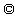1.version 30.9.97, 2.enlarged version 5.10.97
updated 6th of October 97

Abstract:

In this article we prove overunity efficiency of a simple R-L test circuit experimentally and theoretically.

It must be noted, that this test circuit was tried using a bifilar coil, but used as 2 normal in series
wired coils and NOT in BIFILAR NON-INDUCTIVE mode !

As our theory calculation uses 2 normal coils, where the flux does NOT interact, it is advised, that you should just use 2 normal coils which are not magnetically coupled , if you want to try this experiment yourself !

Experimental section:
Following ideas of Fred Epps and Stefan Hartmann (1) using bifilar coils for overunity experiments one of the authors (J.L.N.) built up the following circuit which showed a remarkable waveform at the load resistor R 8 of the circuit.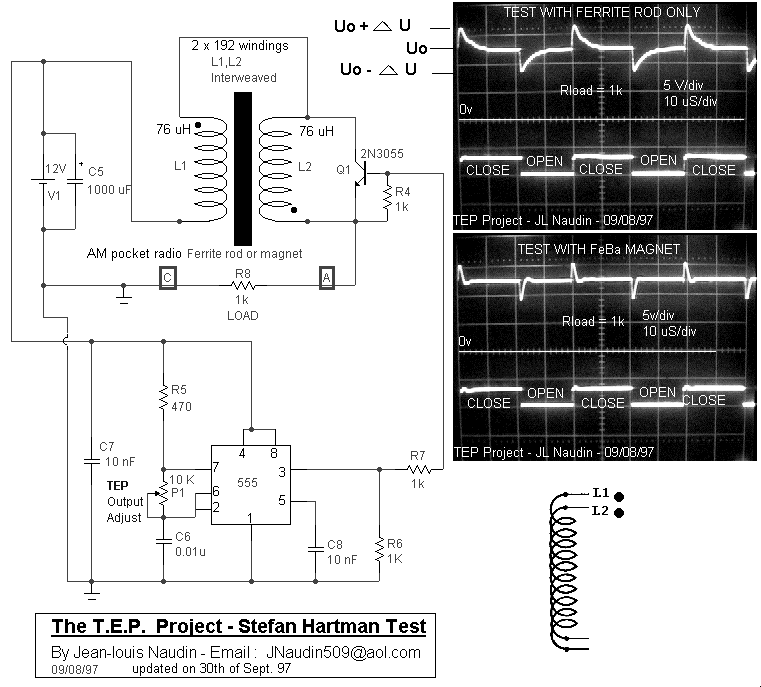Calculation of spent energies:
The overunity efficiency of this current at the load resistor can be seen as follows:
Because the circuit is a R-L circuit principally we can decribe the waveform of each half cycle in the oscillogram at R 8 by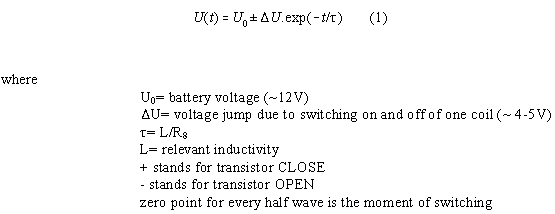Therefrom we can calculate the current in the cycle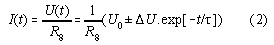Therefrom follows: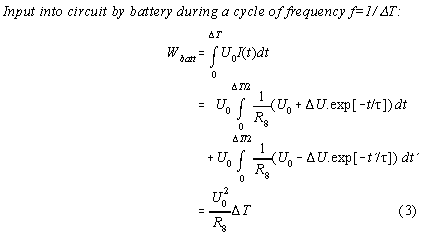Hints for possible improvements of efficiency:
1) It is useful to choose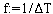so thatbecomes a maximum which can be found by extrapolation by solving the equation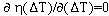. Therefrom we get the condition for maximum efficiency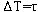. Under this condition the possible maximum efficiency is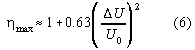2) The voltage jump due to the switching should be maximized. Therefore the switched coil should be big and the switching of the coil as fast as possible. Therefore, the parasitic capacity of the coil should be reduced as far as possible by winding the coil more in heigth than in length like flat Tesla coils which are the extremum of this design.
3) The influence of the timer should be decoupled as far as possible
4) Up spikes enhance efficiency, down spikes depress it. Therefore, the peak of the down spike could be lowered by a slowly switching on of the transistor by an appropriate slowly voltage ramp at the basis of the transistor or FET.

Theoretical section: (uses 2 normal aircore coils, not coupled magnetically !)
In principle the circuit under consideration is a simple R-L circuit which is represented in a simplified form in the picture below. The definition of the variables of analysis below can be taken therefrom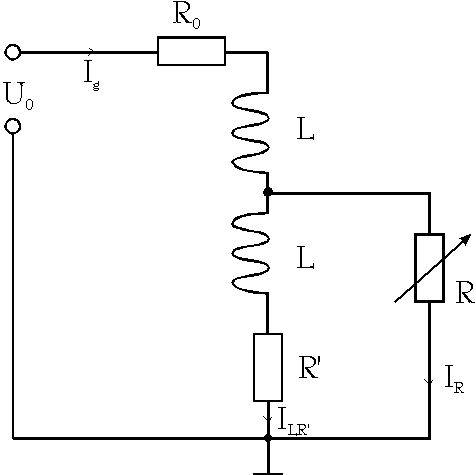The differential equation of this circuit is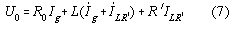Because ILR' = Ig - IR the last equation can be written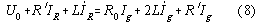The left side of the last equation is the inhomogenic part of the differential equation because it can be set by the basis of the FET or transistor represented in figure above as variable resistor R. We assume for demonstration purposes that IR will be set by FET in the opening phase so that (with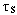as switching time)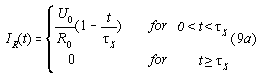for the closing phase holdsFurthermore we choose R' so that R'.I LR'<<U0 , so that R'.I LR' can be neglected against U0 .
Therefore equation (8) can be reduced to a normal R-L circuit subjected to rectangular voltage spikes of heigth with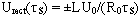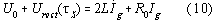The solution of this problem is solved in the most standard textbook of electronics and has a form which resembles exactly the pictures from scope shown above.

Conclusion:
In order to fulfill the law of conservation of energy (first law of thermodynamics) energy must be coming into the circuit at the switched coil.
If this additional energy is converted from surounding-heat then a cooling down of the coil and ferrite should be measurable !
But problems arrive with this explanation if we have aircore coils in vacuum at very low temperatures. A least there other possibilities of explanation like Zero Point Energy (ZPE), G-strain energy, aether or similar concepts have to be taken into account.

Bibliography:
(1) see at mailing list freenrg-l at www.eskimo.com/~billb in the archives for July-September 1997

(2) See the TEP development at Jean Louis Naudin´s site at: members.aol.com/jnaudin509

(3) See the latest TEP pictures here at the site: www.overunity.de/tep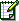Emails : J.L. Naudin , W.D.Bauer, S.Hartmann